Neocijenjeno
Sept. 18, 2023, 9:02 p.m. (3 days, 12 hours)
Let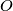$O$ be the circumcenter and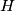$H$ the orthocenter of an acute triangle$ABC.$ Show that there exist points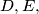$D, E,$ and$F$ on sides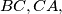$BC,CA,$ and$AB$ respectively such thatand the lines$AD, BE,$ and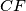$CF$ are concurrent.
Upozorenje: Ovaj zadatak još niste riješili!
Kliknite ovdje kako biste prikazali rješenje.